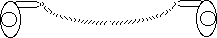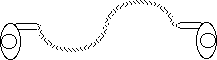The fundamental frequency

I've got rhythm

Introduction

In this exploration we will measure the frequencies of oscillation of the harmonic resonances of a stretched spring. The frequency of the fundamental is approximately the same as the frequency which with a pulse travels from one end of the spring to the other and back again. The frequencies of the higher harmonics are simple integral multiples of the fundamental frequency.

Materials

• 25 feet of coiled phone cord,(heavy-duty cord will last longer)
• a stopwatch

To Do and Notice

You may wish to do the Slow Waves on a Phonecord exploration before you do this one.

Move your hand back and forth to set up a one-hump standing wave.One person moves a hand back and forth a little to create a large motion of the phone cord.

Find the frequency of back and forth motions of your hand at which small motions of your hand grow to large motions of the cord. This is called the fundamental oscillation of the cord.
Measure the period, T, of this oscillation &emdash; the time it takes to go through one complete cycle and return to its original position. Use the stopwatch. You can improve the accuracy of your timing by measuring the time for 10 oscillations and then dividing by ten. Remember to start your count at "ZERO" as you start the stopwatch then to end your count at "TEN."
Compare this to the time it takes for a short pulse to go from one end of the cord to the other and back again.
You can actually establish this one-hump pattern and then send a single short pulse down the cord. Notice that the pulse travels down the cord and returns to your hand in the same time as the large standing wave hump moves to one side then returns to its original position.

These two times theoretically should be the same, and usually are nearly the same.

What's Going On?

Every time you move your hand you are sending a wave down the spring. The wave reflects off the other end and the reflected wave passes through the transmitted wave. Since the wave and its reflection have nearly the same amplitude and period they add to make a standing wave. (see the Hanging Slinky activity.)

Math Root

Frequency and period

The one-hump of the standing wave which fits on the cord is one-half of a sine wave. So the wavelength of the fundamental, L, is twice the length of the cord, 2d.

The frequency, f, is the number of complete cycles of the wave per second. It is the reciprocal of the period.

f = 1/T

For a period of one second the frequency is one cycle per second or one hertz.

Math Root

Frequency of the fundamental

We can predict the frequency of the fundamental, f1 if we know the speed of pulses on the cord, v, and the length of the cord,d.

f1 = 2d/v

There is an important relation for all waves:
the speed of the wave, v, is the product of the frequency, f, and the wavelength, L .

v = fL

Check the units.
Since L = 2d these two relations give the same result.

Higher harmonics

To Do and notice

You can make a two hump pattern on the cord. This pattern of motion is known as the second harmonic. In the second harmonic, one complete wave fits on the cord. L = d. Notice that it has a node in the middle, a point with no side to side motion.Make a two-hump pattern on the spring.

Measure the period of this wave.
The period will be close to 1/2 of the period of the fundamental.
The frequency f
2 will be double the frequency of the fundamental.

Optional ways to compare the frequencies of the second harmonic and the fundamental. You can time the time it takes for the fundamental to make 5 oscillations and compare it to the time it takes the second harmonic to make ten oscillations.

You can also count the beat of the fundamental, one-two-three...counting each time the cord hits the leftmost edge of its travel.
Then count the beat of the second harmonic, one-two-three ... And finally count every other beat of the second harmonic. Counting every other maximum of the second harmonic should give the same frequency as counting every maximum of the fundamental.

If you have two phone cords you can have them side by side. While one is made to oscillate in the fundamental, the other is made to oscillate in the second harmonic. The second harmonic can be seen to be twice the frequency of the fundamental.

Math Root

Harmonic Frequencies

The frequency of the second harmonic is twice that of the fundamental,
exactly one wave fits on the cord
so L = d and
f
2 = 2f1 = L2/v = d/v.

Measure the frequency of the third harmonic, which has 3 humps, or one and a half waves, on the spring. Notice the 2 nodes. The frequency is 3 times the frequency of the fundamental.

f3 = 3 f1

See how many harmonics you can make and measure. Our record is seven. For harmonic number n the frequency is

fn= n f1.

The higher frequency harmonics are harder to make than the low frequency harmonics. The amount of energy contained in a harmonic increases like the square of the frequency. So the fifth harmonic takes 25 times as much work to make as a fundamental frequency oscillation with the same amplitude.

You can also make two resonances on the spring at the same time. Try setting up a third harmonic and then get the three humped pattern moving back and forth in a one humped pattern at the same time. In fact the spring can vibrate to many different resonance frequencies at the same time. But it takes some practice to be able to generate more than one.

What's going on?

Why are the resonant frequencies of the cord simply integer multiples of the fundamental? When you move your hand you are sending a pulse down the cord, if you wait for the pulse to travel down the cord and return to your hand, then add another pulse to it, the pulse will grow. This is what you are doing when you build up a the fundamental resonance of the cord, adding energy to one pulse as it travels back and forth along the cord.
In the second harmonic you have two pulses on the cord. The harmonic grows when you move your hand with a rhythm precisely matching the time it takes pulses to move down the spring and arrive back at your hand. With two pulses on the cord you must move your hand at twice the frequency as when one pulse is on the cord. There is no way to have a fractional number of pulses on the cord. So only integer multiples of the fundamental frequency will grow to resonance. (Note that the pulses are required to be evenly spaced, this requirement comes from the fact that they are sine waves and sine waves have uniformly spaced maxima.)